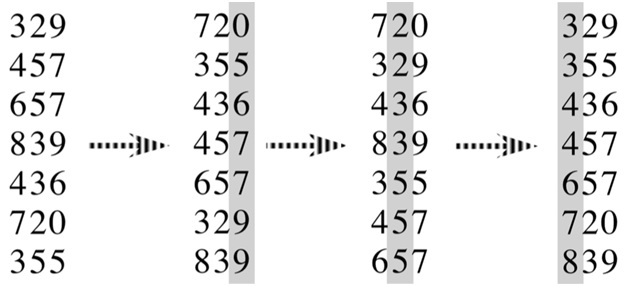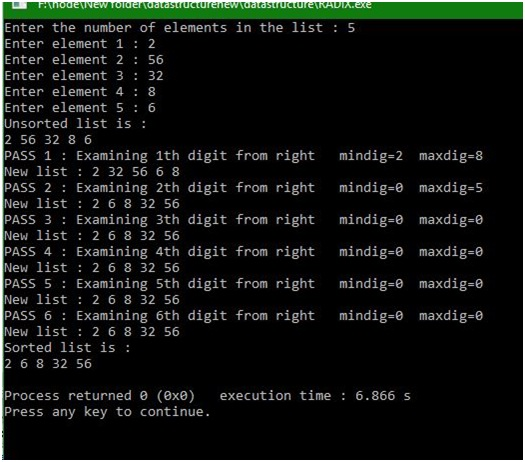# What is radix sort, why it is called non-comparative integer sorting?

Submitted by Manu Jemini, on January 18, 2018

When we say sorting it means serializing a collection of data inside any the collections like arrays, linked list etc. There is one good reason for implementing sorting on any collection is that it makes the searching very fast as compared to the collection which is unsorted. Now, this is not true for every test case but on an average, it is so.So what is Radix sort? Well, it is very simple to learn what it is about. In the Radix sort, the control makes passes all over the array equal to the number of the digits of the maximum integer. In the case above the maximum integer have three digits so the algorithm will make the three passes in the array.

In the First pass, the algorithm will sort the elements on the basis of the first digit. For example, let’s take two digits 121 and 109. During the first pass, the integer 109 will be treated as the bigger number than the 121 because the first digit from the left is 9 and 1 for 109 and 121 respectively.

In the second pass, the algorithm will sort the elements on the basis of the second digit. For example, let’s take two digits 121 and 109. During the first pass, the integer 109 will be treated as the smaller number than the 121 because the second digit from the left is 2 and 0 for 109 and 121 respectively.

As the third, digit is same for both integers the number 121 will remain as the bigger integer over 109.

## C program for radix sort

```# include<stdio.h>
# include<malloc.h>

struct  node
{
int info ;
}*start=NULL;

void display()
{
struct node *p=start;
while( p !=NULL)
{
printf("%d ", p->info);
}
printf("\n");
}/*End of display()*/

/*This function returns kth digit of a number*/
int digit(int number, int k)
{
int digit, i ;
for(i = 1 ; i <=k ; i++)
{
digit = number % 10 ;
number = number /10 ;
}
return(digit);
}/*End of digit()*/

/* This function finds number of digits in the largest element of the list */
int large_dig()
{
struct node *p=start ;
int large = 0,ndig = 0 ;

while(p != NULL)
{
if(p ->info > large)
large = p->info;
}
printf("Largest Element is %d , ",large);
while(large != 0)
{
ndig++;
large = large/10 ;
}

printf("Number of digits in it are %d\n",ndig);
return(ndig);
} /*End of large_dig()*/

{
int i,k,dig,maxdig,mindig,least_sig,most_sig;
struct node *p, *rear, *front;

least_sig=1;

for(k = least_sig; k <= most_sig ; k++)
{
printf("PASS %d : Examining %dth digit from right   ",k,k);
for(i = 0 ; i <= 9 ; i++)
{
rear[i] = NULL;
front[i] = NULL ;
}
maxdig=0;
mindig=9;
p = start ;
while( p != NULL)
{
/*Find kth digit in the number*/
dig = digit(p->info, k);
if(dig>maxdig)
maxdig=dig;
if(dig<mindig)
mindig=dig;

/*Add the number to queue of dig*/
if(front[dig] == NULL)
front[dig] = p ;
else
rear[dig] = p ;
p=p->link;/*Go to next number in the list*/
}/*End while */
/* maxdig and mindig are the maximum amd minimum
digits of the kth digits of all the numbers*/
printf("mindig=%d  maxdig=%d\n",mindig,maxdig);
/*Join all the queues to form the new linked list*/
start=front[mindig];
for(i=mindig;i<maxdig;i++)
{
if(rear[i+1]!=NULL)
else
rear[i+1]=rear[i];
}
printf("New list : ");
display();
}/* End for */

main()
{
struct node *tmp,*q;
int i,n,item;

printf("Enter the number of elements in the list : ");
scanf("%d", &n);

for(i=0;i<n;i++)
{
printf("Enter element %d : ",i+1);
scanf("%d",&item);

/* Inserting elements in the linked list */
tmp= (struct node *)malloc(sizeof(struct node));
tmp->info=item;

if(start==NULL) /* Inserting first element */
start=tmp;
else
{
q=start;
}
}/*End of for*/

printf("Unsorted list is :\n");
display();
printf("Sorted list is :\n");
display ();
}/*End of main()*/
```

Output